# Apples and pears

Apples cost 50 cents piece, pears 60 cents piece, bananas cheaper than pears. Grandma bought 5 pieces of fruit. There was only one banana, and I paid 2 euros 75 cents. How many apples and how many pears?

j1 =  0
h1 =  4
j2 =  1
h2 =  3
j3 =  2
h3 =  2

### Step-by-step explanation:

${h}_{1}=5-1-{j}_{1}=5-1-0=4$
${j}_{2}=1=1$
${h}_{2}=5-1-{j}_{2}=5-1-1=3$
${j}_{3}=2=2$
${h}_{3}=5-1-{j}_{3}=5-1-2=2$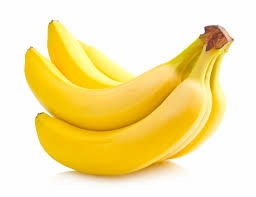Did you find an error or inaccuracy? Feel free to write us. Thank you!Tips to related online calculators
Do you solve Diofant problems and looking for a calculator of Diofant integer equations?

## Related math problems and questions:

• Sales of productsFor 80 pieces of two quality products a total sales is 175 Eur. If the first quality product was sold for n EUR per piece (n natural number) and the second quality product after 2 EUR per piece. How many pieces of the first quality were sold?
• The bun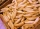The bun costs CZK 2 and the rolls CZK 3.5. Hanka bought 22 pieces of bread, bought only buns and rolls and paid CZK 65. how many rolls did she buy and how many rolls?
• Sale offProduct cost 95 euros before sale off. After sale off cost 74 euros and 10 cents. About what percentage of produt became cheaper?In six baskets, the seller has fruit. In individual baskets, there are only apples or just pears with the following number of fruits: 5,6,12,14,23 and 29. "If I sell this basket," the salesman thinks, "then I will have just as many apples as a pear." Whic
• Car rent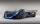Rental cars are paid a fixed daily fee plus 16 cents for each kilometer traveled. Samuel want to rent a car for a day and worked out that will pay 175 euros. When paying he got a 20% discount on the daily fee, the cost per kilometer is unchanged. Samuel t
• TreesAlong the road were planted 250 trees of two types. Cherry for 60 CZK apiece and apple 50 CZK apiece. The entire plantation cost 12,800 CZK. How many was cherries and apples?
• They boughtThey bought the students a set of notebooks for 65 euros. The first set cost € 2 and the second € 3. There were 5 times as many two-euro sets as three-set ones. How many sets of workbooks are there?
• Coins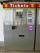In the ticket machine were together one hundred coins. They were only 20 and 50 cent coins. The sum total was 29 euros and 60 cents. How many were in ticket machine coins, and which type?
• Special watchFero bought a special watch on the market. They have only one (minute) hand and a display that shows which angle between the hour and minute hand. How many hours was his watch shown - the minute hand points to number 2; the display shows 125°?
• Three friends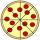Divide 570 euros to three friends so that first will get 50 euros less than the second and third twice more than the first. How many euros will get everyone?
• Seamstress 2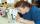Seamstress bought two kinds of textile in whole meters. One at 50 SKK and the second 70 SKK per meter. How many meter bought from this two textiles when paid totally 1540 SKK?
• SeamstressSeamstress bought two kinds of textile together 25 meters. One at 50 SKK and the second 70 SKK per meter. How many meter bought from this two textiles when paid totally 1540 SKK?
• Five-digit numberAnna thinks of a five-digit number that is not divisible by three or four. If he increments each digit by one, it gets a five-digit number that is divisible by three. If he reduces each digit by one, he gets a five-digit number divisible by four. If it sw
• Apples 14Maya bought 18 apples. She used 50% of the apples to make apple pies, and 1/3 of the apples were mixed with other fruits to make the salad. How many apples were left?
• Two math problems1) The sum of twice a number and -6 is nine more than the opposite of that number. Find the number. 2) A collection of 27 coins, all nickels, and dimes, is worth \$2.10. How many of each coin are there? The dime, in United States usage, is a ten-cent coin.
• AlcoholHow many 55% alcohol we need to pour into 14 liters 75% alcohol to get p3% alcohol? How many 65% alcohol we get?
• PaintingThe room is long 50 meters and wide 60dm and 300 cm high. Calculate how much it will cost painting if area of windows and doors is 15% of the total area. One square meter cost 50cents.